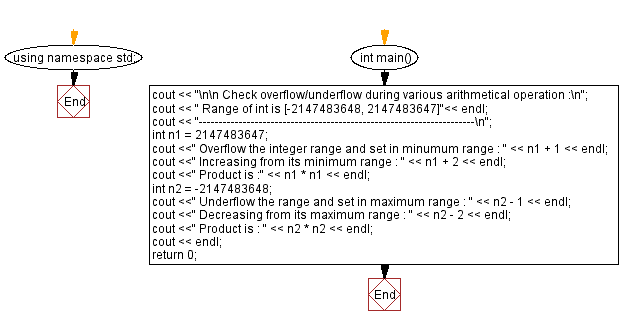﻿ C++ : Check overflow/underflow during various operation

# C++ Exercises: Check overflow/underflow during various arithmetical operation

## C++ Basic: Exercise-8 with Solution

Write a C++ program to check overflow/underflow during various arithmetic operations.

Sample Solution:

C++ Code :

``````#include <iostream>
using namespace std;

int main()
{
cout << "\n\n Check overflow/underflow during various arithmetical operation :\n";
cout << " Range of int is [-2147483648, 2147483647]"<< endl;
cout << "---------------------------------------------------------------------\n";
int n1 = 2147483647;      // maximum range of integer
cout <<" Overflow the integer range and set in minimum range : " << n1 + 1 << endl;
cout <<" Increasing from its minimum range : " << n1 + 2 << endl;
cout <<" Product is :" << n1 * n1 << endl;

int n2 = -2147483648;     // minimum range of integer
cout <<" Underflow the range and set in maximum range : " << n2 - 1 << endl;
cout <<" Decreasing from its maximum range : " << n2 - 2 << endl;
cout <<" Product is : " << n2 * n2 << endl;
cout << endl;
return 0;
}
``````

Sample Output:

``` Check overflow/underflow during various arithmetical operation :
Range of int is [-2147483648, 2147483647]
---------------------------------------------------------------------
Overflow the integer range and set in minimum range : -2147483648
Increasing from its minimum range : -2147483647
Product is :1
Underflow the range and set in maximum range : 2147483647
Decreasing from its maximum range : 2147483646
Product is : 0
```

Flowchart:C++ Code Editor:

What is the difficulty level of this exercise?

﻿

## C++ Programming: Tips of the Day

Why is there no std::stou?

The most pat answer would be that the C library has no corresponding "strtou", and the C++11 string functions are all just thinly veiled wrappers around the C library functions: The std::sto* functions mirror strto*, and the std::to_string functions use sprintf.

Ref: https://bit.ly/3wtz2qA

We are closing our Disqus commenting system for some maintenanace issues. You may write to us at reach[at]yahoo[dot]com or visit us at Facebook